•5星
135.91MB qq_34351067 2021-04-16 14:11:28
•5星
14.03MB qq_41934573 2021-05-26 22:12:39
•5星
326KB weixin_42120750 2021-04-26 16:06:08
•5星
403KB alongiii 2021-01-04 10:40:20
•5星
59.58MB Wanjunjie812 2021-04-30 00:46:00
•5星
1KB weixin_42665725 2021-09-28 21:36:00
•5星
157.64MB Wanjunjie812 2021-01-07 17:02:34
•5星
3.52MB wenyusuran 2021-03-26 09:54:09
•5星
295KB weixin_42696271 2021-09-11 10:16:50
•5星
2KB weixin_45479707 2021-06-30 14:17:26
• MATLAB坐标提取 MATLAB

2KB sdkdgongbin 2018-12-11 17:04:37
• 利用matlab从图片中提取曲线坐标数据 matlab

此时，如果能从文中把这幅图截取下来，输入到一个函数中去，最后能返回从图片中提取到的曲线的坐标数据，岂不美哉。这便是本文的工作。 1.思路详解与分析 1.1准备待提取数据的曲线图片 将待提取数据的曲线的图片...

目录0.引言

在读文献的时，经常遇到这样的情况：文章里提出的方法好有趣啊，好想拿文中用的数据来试试看看能不能得到相近的结果，可是文中只有根据原始数据绘制的曲线图，没有数据。如下图所示。此时，如果能从文中把这幅图截取下来，输入到一个函数中去，最后能返回从图片中提取到的曲线的坐标数据，岂不美哉。这便是本文的工作。

1.思路详解与分析

1.1准备待提取数据的曲线图片

1.2曲线图片预处理与数据转换

曲线图片预处理步骤的主要工作包含如下：

（1）图像二值化

将输入图像进行二值化处理，但分割得到的结果并不全为数据，其中可能还包括坐标轴等干扰点需要去除。

（2）获取从图片像素到曲线坐标的定标数据

首先，通过ginput()手动从图片中提取到两个像素点，这两个点分别为曲线坐标框的左上角和右下角。此时,便获得了曲线在图片上的像素范围

[x_index_min, x_index_max] & [y_index_min, y_index_max]

然后，手动输入实际曲线的数据坐标范围 [x_min, x_max] & [ymin, y_max_]. 如下所示。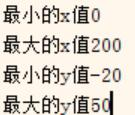此时，一方面得到了像素坐标，一方面得到了实际坐标。接下来便利用这对数据，将图片中全部的像素坐标转换到实际坐标。

最终，得到了由图片提取到的数据散点图，如下:可以看到，此时得到的结果，虽然曲线与所需要的相近，但曲线外的部分，如坐标轴框、坐标轴刻度等也被转换成了数据，还需要进一步的处理。

1.3数据的进一步处理并得到最终曲线

这一步的主要工作是在数据中除去曲线之外的部分（包括坐标轴框、坐标轴刻度等）；以及解决一个x数据对应多个y数据的情况。

显然，坐标轴在整幅数据中，均处于边界位置，因此，很容易想到的一种方法是，设定阈值，将距离边界较近的数据直接删除。这里，设定了两个阈值，一个用来限定x方向上的数据，一个用来限定y方向上的数据。比如设定：rate_x=0.08; rate_y=0.05; 意思是阈值设定为曲线最前端8%和最后段8%的数据，曲线最顶端5%和最底端5%的数据。

进一步的，对于提取到的数据图，大多数情况一个x会对应若干y，因为数据是由图片转化而来，而图片的分辨率有限，一个实际数据点会用多个像素来表示。解决此问题的中心思想是将同一个x对应的若干个y取均值，但不能直接求均值，因为还有很多y是噪声(如坐标轴线、由图片噪声带入的干扰点等)需要先去除，在第一个问题中，通过限定y的范围，已经在一定程度上除去了部分干扰，在此基础上，我们求取一个x对应的这组y值的均值mean与标准差std，当某些y值位于[mean-std , mean+std]之外，则认为这些y值波动太大，将它们删去。

到这里，我们就将数据的处理部分基本完成了，我们将处理后的数据再次绘制成曲线，便可以得到如下对比处理之前的数据，由于限定了范围，因此曲线图片中带来的坐标框等内容转化而来的数据已经被删去。

将需要提取坐标的曲线图片，和我们提取并处理后的数据绘制的曲线，放在一起对比如下：可以看到，与原曲线图片相比，提取到的数据曲线相似度能达到较高要求。但进一步观察会发现，右图曲线较左图而言，高频分量有一定的减少（即右图曲线更平滑），原因在于数据处理时，对同一个x对应的这组y值进行了均值处理，则在图像上近似反应为均值滤波，从而使得提取到的数据绘制成的曲线的高频分量被滤除。

最后，将提取到的最终数据，保存起来如下：1.4进一步的讨论——曲线拟合

通过对图片中曲线的数据提取，可以得到数值上的答案，这会带来进一步的思考，即能否得到这些数据的解析表达式。很容易想到，利用最小二乘法来拟合这些数据，这便涉及到了曲线的拟合。（插值与拟合可以这么理解：对于数据点集，若均落在曲线上，则该曲线为插值曲线，否则为拟合曲线）

对于一些简单的曲线图片(如下)，可以考虑用泰勒级数来近似，即多项式拟合。数据提取并拟合的结果展示如下同时还能得到拟合多项式的系数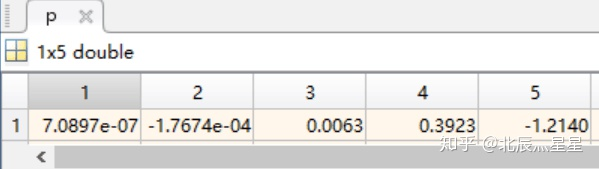从而得到该曲线的多项式(这里采用四阶多项式)表达式为:理论上，泰勒级数可以分解任何函数，但实际上，多项式拟合的次数太高，会出现龙格库塔现象，即摆尾现象。因此，多项式拟合的阶数不易过高，一般低于5阶。对于本文最开始的那幅曲线而言，仅5阶的泰勒级数就显得力不从心了，因此，对于这种存在波动剧烈的函数，可以考虑用傅里叶级数进行拟合，或者如果能提供先验知识，可以直接用先验表达式进行拟合。

在MATLAB中，提供了cftool工具箱，其提供了拟合与插值的GUI，使用非常方便，直接在命令窗输入cftool即可调用，cftool界面如下所示，其具体使用方法不在此赘述。2.MATLAB程序

MATLAB源代码如下所示，和以往的风格一样，提供了详细的注释

% //提取图片中的曲线数据
clear,clc,close all
%% //图片与曲线间的定标
im=rgb2gray(im); %//灰度变化
thresh = graythresh(im); %//二值化阈值
im=im2bw(im,thresh); %//二值化
set(0,'defaultfigurecolor','w')
imshow(im) %//显示图片
[y,x]=find(im==0); %//找出图形中的“黑点”的坐标。该坐标是一维数据。
y=max(y)-y; %//将屏幕坐标转换为右手系笛卡尔坐标
y=fliplr(y); %//fliplr()——左右翻转数组
plot(x,y,'r.','Markersize', 2);
disp('请在Figrure中先后点击实际坐标框的两个顶点(左上点和右下点)，即A、B两点. ');
[Xx,Yy]=ginput(2); %//Xx,Yy——指实际坐标框的两个顶点
min_x=input('最小的x值');  %//输入x轴最小值
max_x=input('最大的x值');  %//输入x轴最大值
min_y=input('最小的y值');  %//输入y轴最小值
max_y=input('最大的y值');  %//输入y轴最大值
x=(x-Xx(1))*(max_x-min_x)/(Xx(2)-Xx(1))+min_x;
y=(y-Yy(1))*(min_y-max_y)/(Yy(2)-Yy(1))+max_y;
plot(x,y,'r.','Markersize', 2);
axis([min_x,max_x,min_y,max_y])  %//根据输入设置坐标范围
title('由原图片得到的未处理散点图')
%% //将散点转换为可用的曲线
%//需处理的问题与解决思路
%//(1)散点图中可能一个x对应好几个y <---> 保留mean()-std()到mean()+std()之间的y值 并取平均处理
%//(2)曲线的最前端和最后段干扰较大 <---> 去掉曲线整体的前(如5%)和后5%
%//(3)曲线的最顶端和最底段干扰较大 <---> 去掉曲线整体的上10%和下10%

%//参数预设
rate_x=0.08;  %//曲线的最前端和最后段删除比例
rate_y=0.05;  %//曲线的最顶端和最底段删除比例

[x_uni,index_x_uni]=unique(x);  %//找出有多少个不同的x坐标

x_uni(1:floor(length(x_uni)*rate_x))=[];  %//除去前rate_x(如5%)的x坐标
x_uni(floor(length(x_uni)*(1-rate_x)):end)=[];  %//除去后rate_x的x坐标
index_x_uni(1:floor(length(index_x_uni)*rate_x))=[];  %//除去前rate_x的x坐标
index_x_uni(floor(length(index_x_uni)*(1-rate_x)):end)=[];  %//除去后rate_x的x坐标

[mxu,~]=size(x_uni);
[mx,~]=size(x);
for ii=1:mxu
if ii==mxu
ytemp=y(index_x_uni(ii):mx);
else
ytemp=y(index_x_uni(ii):index_x_uni(ii+1));
end
% //删除方差过大的异常点
threshold1=mean(ytemp)-std(ytemp);
threshold2=mean(ytemp)+std(ytemp);
ytemp(find(ytemp<threshold1))=[];  %//删除同一个x对应的一段y中的异常点
ytemp(find(ytemp>threshold2))=[];
% //删除距顶端和底端较近的点
thresholdy=(max_y-min_y)*rate_y;  %//y坐标向阈值
ytemp(find(ytemp>max_y-thresholdy))=[];  %//删除y轴向距离顶端与底端距离小于rate_y的坐标
ytemp(find(ytemp<min_y+thresholdy))=[];
% //剩下的y求均值
y_uni(ii)=mean(ytemp);
end
% //此时很多x_uni点处对应的y_uni为空,即NAN,要进一步删去这些空点
x_uni(find(isnan(y_uni)))=[];
y_uni(find(isnan(y_uni)))=[];
% //画图
figure,plot(x_uni,y_uni),title('经处理后得到的扫描曲线')
axis([min_x,max_x,min_y,max_y])  %//根据输入设置坐标范围
% //将最终提取到的x与y数据保存
curve_val(1,:)=x_uni';
curve_val(2,:)=y_uni;
%% //对提取出的数据进行拟合(按实际情况进行修改)
[p,s]=polyfit(curve_val(1,:),curve_val(2,:),4);  %//多项式拟合(为避免龙格库塔,多项式拟合阶数不宜太高)
[y_fit,DELTA]=polyval(p,x_uni,s);  %//求拟合后多项式在x_uni对应的y_fit值
figure,plot(x_uni,y_fit),title('拟合后的曲线')
axis([min_x,max_x,min_y,max_y])  %//根据输入设置坐标范围

转自知乎专栏

展开全文weixin_43964993 2020-08-25 14:09:08
• 501B qq_22788061 2020-09-28 09:04:34
•5星
2KB weixin_42696271 2021-09-10 17:54:25
• 3KB yan13478760131 2018-06-03 17:39:33
• CODE:function varargout = ginput_ax_mod2(ha,n)if nargin<2n=1;endk = 0;button = 0;%%// Tolerance so that in the inifnity case, this could act as%%// the thresholding distance below which the%%// inp...

CODE:

function varargout = ginput_ax_mod2(ha,n)

if nargin<2

n=1;

end

k = 0;

button = 0;

%%// Tolerance so that in the inifnity case, this could act as

%%// the thresholding distance below which the

%%// input extracting operation must be terminated

TOL = 0.01;

%%// Placeholders for X-Y and button type could be stored

button1 = [];

xy = [];

hf = get(ha,'parent');

figure(hf);

set(hf,'WindowButtonMotionFcn',@changepointer)

set(ha,'ButtonDownFcn',@getpoints)

hp = get(ha,'children');

ht = get(hp,'hittest');

set(hp,'hittest','off')

axlim = get(ha,'Position');

fglim = get(hf,'Position');

x1 = axlim(1)*fglim(3) + fglim(1);

x2 = (axlim(1)+axlim(3))*fglim(3) + fglim(1);

y1 = axlim(2)*fglim(4) + fglim(2);

y2 = (axlim(2)+axlim(4))*fglim(4) + fglim(2);

waitfor(hf,'WindowButtonMotionFcn',[])

if iscell(ht)

for jj=1:length(ht)

set(hp(jj),'hittest',ht{jj})

end

else

set(hp,'hittest',ht)

end

selType = get(hf,'SelectionType');

% Mouse-Button recognition...

if(strcmp(button, 'normal'))

button = 1; % left

elseif(strcmp(button, 'extend'))

button = 2; % right

elseif(strcmp(button, 'alt'))

button = 3; % middle

else

button = 4; % double click any mousebutton

end

if nargout==3

varargout{1} = xy(:,1);

varargout{2} = xy(:,2);

varargout{3} = button1(:,1);

elseif nargout==2

varargout{1} = xy(:,1);

varargout{2} = xy(:,2);

else

varargout{1} = xy;

end

function changepointer(~,~)

pntr = get(0,'PointerLocation');

if pntr(1)>x1 && pntr(1)y1 && pntr(2)

set(hf,'Pointer','crosshair')

else

set(hf,'Pointer','arrow')

end

end

function getpoints(src,evnt)

cp = get(src,'CurrentPoint');

button = get(hf, 'SelectionType');

k = k+1;

if k==1

xy = [xy ;cp(1,1:2)];

button1 = [button1; {button}];

end

if k>=2

if pdist2(cp(1,1:2),xy(k-1,:))

k = n;

else

xy = [xy ;cp(1,1:2)];

button1 = [button1; {button}];

end

end

if k==n

set(hf,'Pointer','arrow')

set(hf,'WindowButtonMotionFcn',[])

set(ha,'ButtonDownFcn',[])

return;

end

end

end

展开全文weixin_39997795 2021-04-20 15:25:51
• 0.引言 在读文献的时，经常遇到这样的情况：文章里提出的方法好有趣啊，好想拿文中...此时，如果能从文中把这幅图截取下来，输入到一个函数中去，最后能返回从图片中提取到的曲线的坐标数据，岂不美哉。 2.MATLAB程...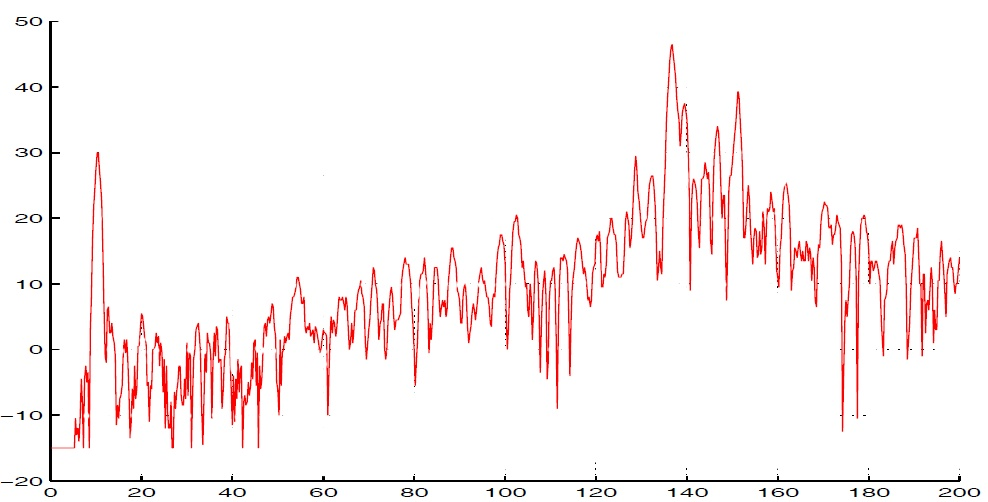转载: https://zhuanlan.zhihu.com/p/52112012
0.引言
在读文献的时，经常遇到这样的情况：文章里提出的方法好有趣啊，好想拿文中用的数据来试试看看能不能得到相近的结果，可是文中只有根据原始数据绘制的曲线图，没有数据。如下图所示。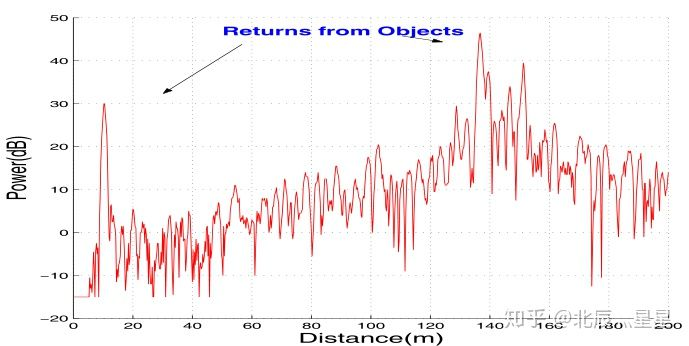此时，如果能从文中把这幅图截取下来，输入到一个函数中去，最后能返回从图片中提取到的曲线的坐标数据，岂不美哉。

2.MATLAB程序

MATLAB源代码如下所示，和以往的风格一样，提供了详细的注释

% 提取图片中的曲线数据
clear,clc,close all
%% 图片与曲线间的定标
im=rgb2gray(im);%灰度变化
thresh = graythresh(im);%二值化阈值
im=im2bw(im,thresh);%二值化
set(0,'defaultfigurecolor','w')
imshow(im)%显示图片
[y,x]=find(im==0);%找出图形中的“黑点”的坐标。该坐标是一维数据。
y=max(y)-y;%将屏幕坐标转换为右手系笛卡尔坐标
y=fliplr(y);%fliplr()——左右翻转数组
plot(x,y,'r.','Markersize', 2);
disp('请在Figrure中先后点击实际坐标框的两个顶点(左上点和右下点)，即A、B两点. ');
[Xx,Yy]=ginput(2);%Xx,Yy——指实际坐标框的两个顶点
min_x=input('最小的x值');%输入x轴最小值
max_x=input('最大的x值');%输入x轴最大值
min_y=input('最小的y值');%输入y轴最小值
max_y=input('最大的y值');%输入y轴最大值
x=(x-Xx(1))*(max_x-min_x)/(Xx(2)-Xx(1))+min_x;
y=(y-Yy(1))*(min_y-max_y)/(Yy(2)-Yy(1))+max_y;
plot(x,y,'r.','Markersize', 2);
axis([min_x,max_x,min_y,max_y])%根据输入设置坐标范围
title('由原图片得到的未处理散点图')
%% 将散点转换为可用的曲线
%需处理的问题与解决思路
%(1)散点图中可能一个x对应好几个y <---> 保留mean()-std()到mean()+std()之间的y值 并取平均处理
%(2)曲线的最前端和最后段干扰较大 <---> 去掉曲线整体的前(如5%)和后5%
%(3)曲线的最顶端和最底段干扰较大 <---> 去掉曲线整体的上10%和下10%

%参数预设
rate_x=0.08;%曲线的最前端和最后段删除比例
rate_y=0.05;%曲线的最顶端和最底段删除比例

[x_uni,index_x_uni]=unique(x);%找出有多少个不同的x坐标

x_uni(1:floor(length(x_uni)*rate_x))=[];%除去前rate_x(如5%)的x坐标
x_uni(floor(length(x_uni)*(1-rate_x)):end)=[];%除去后rate_x的x坐标
index_x_uni(1:floor(length(index_x_uni)*rate_x))=[];%除去前rate_x的x坐标
index_x_uni(floor(length(index_x_uni)*(1-rate_x)):end)=[];%除去后rate_x的x坐标

[mxu,~]=size(x_uni);
[mx,~]=size(x);
for ii=1:mxu
if ii==mxu
ytemp=y(index_x_uni(ii):mx);
else
ytemp=y(index_x_uni(ii):index_x_uni(ii+1));
end
%删除方差过大的异常点
threshold1=mean(ytemp)-std(ytemp);
threshold2=mean(ytemp)+std(ytemp);
ytemp(find(ytemp<threshold1))=[];%删除同一个x对应的一段y中的异常点
ytemp(find(ytemp>threshold2))=[];
%删除距顶端和底端较近的点
thresholdy=(max_y-min_y)*rate_y;%y坐标向阈值
ytemp(find(ytemp>max_y-thresholdy))=[];%删除y轴向距离顶端与底端距离小于rate_y的坐标
ytemp(find(ytemp<min_y+thresholdy))=[];
%剩下的y求均值
y_uni(ii)=mean(ytemp);
end
%此时很多x_uni点处对应的y_uni为空,即NAN,要进一步删去这些空点
x_uni(find(isnan(y_uni)))=[];
y_uni(find(isnan(y_uni)))=[];
%画图
figure,plot(x_uni,y_uni),title('经处理后得到的扫描曲线')
axis([min_x,max_x,min_y,max_y])%根据输入设置坐标范围
% 将最终提取到的x与y数据保存
curve_val(1,:)=x_uni';
curve_val(2,:)=y_uni;
%% 对提取出的数据进行拟合(按实际情况进行修改)
[p,s]=polyfit(curve_val(1,:),curve_val(2,:),4);%多项式拟合(为避免龙格库塔,多项式拟合阶数不宜太高)
[y_fit,DELTA]=polyval(p,x_uni,s);%求拟合后多项式在x_uni对应的y_fit值
figure,plot(x_uni,y_fit),title('拟合后的曲线')
axis([min_x,max_x,min_y,max_y])%根据输入设置坐标范围

展开全文xuzhexing 2019-06-01 15:13:41
• 526B ww956923110 2019-07-16 21:15:00
• 基于MATLAB提取图片曲线的坐标值（简易GetDada）的实现 matlab 坐标提取 图像处理 图像识别

同事让我帮忙处理一些图片曲线，把图片里的曲线坐标搞出来。 我后悔以前不该乱吹牛。我之前确实搞过一个这样的程序，只不过那个程序更像一个探索性的...图像初步识别的时候，是没有坐标基准的，要在原图里放两个知.

同事让我帮忙处理一些图片曲线，把图片里的曲线坐标搞出来。

我后悔以前不该乱吹牛。我之前确实搞过一个这样的程序，只不过那个程序更像一个探索性的东西，用起来限制很多，稍显鸡肋。

我当时的程序，需要先经过3个步骤的准备，才能开始程序识别坐标。大概的步骤如下：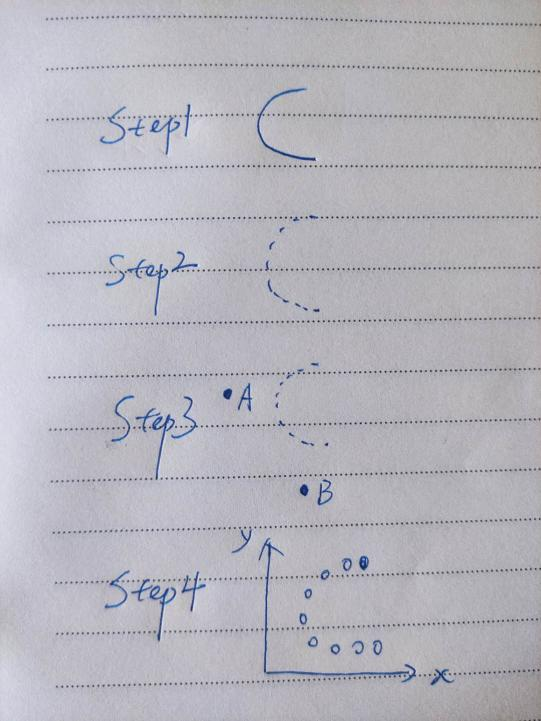Step1. 清场。如果一个图里有很多曲线，要把其他曲线清掉（画图板）；

Step2. 断点。如果曲线是连续的，则要把他们分成一个个小段（图板go on）；

Step3. 定参考点。图像初步识别的时候，是没有坐标基准的，要在原图里放两个知道坐标的点，用于基准定义；

Step4. 程序运行。程序识别出每一个断点的中心位置坐标。

我大概尝试下，这个流程走下来和直接用线程的GetData软件结果差不多。上面几个步骤里面，前两个步骤耗时比较长。

前年冬天在哈尔滨出差的时候，我对step1和step2尝试过自动化的方法。当时我的方法是这样的：

1）针对step1。我搞出了颜色识别算法，对于一个图好多个颜色的线，能够做到提取特定颜色；

2）针对step2。我的设想是，自动在图里画出白色的竖线或者横线，做到对现有曲线的自动分割。

尝试之后，step2的改进失败。这是因为，如果只画横线或者竖线再或者同时画，如果线定的太密，原有曲线被遮挡严重，丢失信息。如果画的太稀疏，则搞得精度不够。这个度对于不同的图，很难有统一的设定，因此实际用起来意义不大。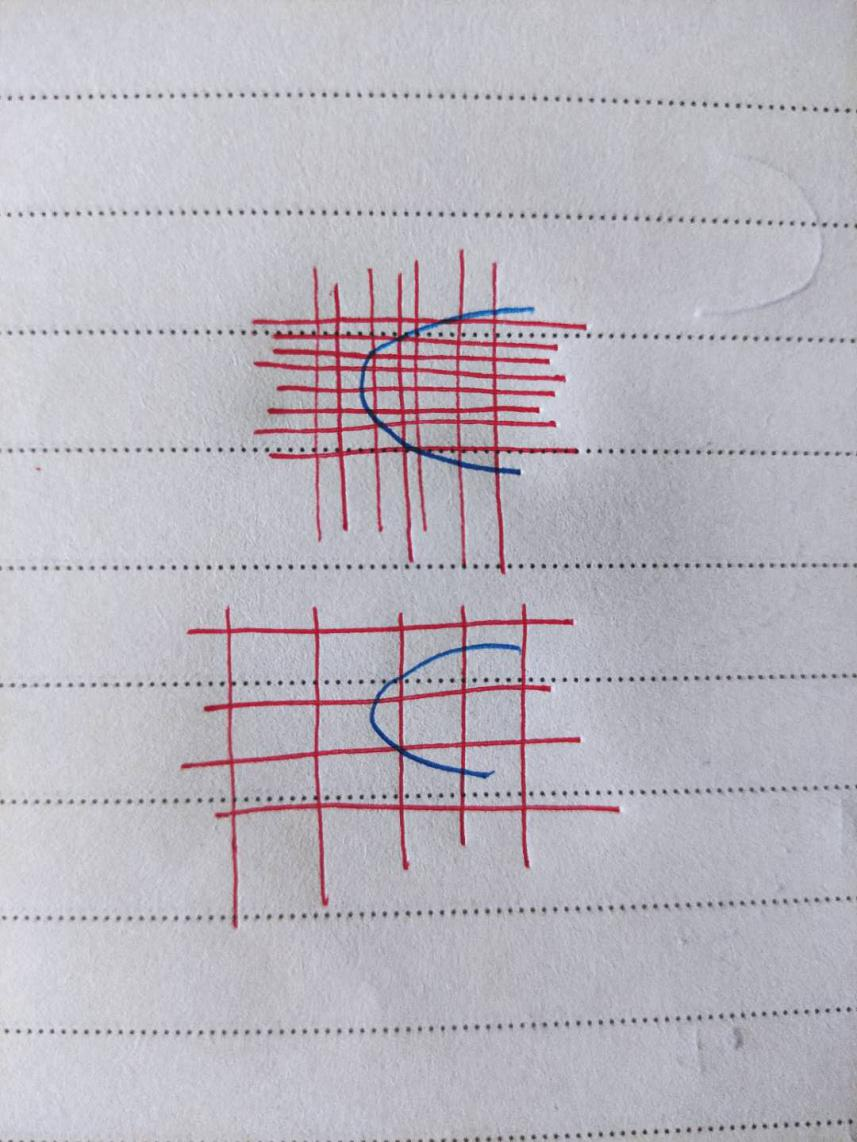如果说一两个图，我用GetData就能很快处理了，一世英名得以保全。拿到手一看，几十个图，每个图2分钟也要搞两三个小时。两三个小时的重复取点工作，光想想，我就觉得自己眼睛会瞎。

我决定砍柴之前再磨一次刀，尝试解决两年前的问题。

1 Step2的解决

实际上，我仔细想了下，之所以出现step2断点操作，主要是我之前的程序里要识别一个封闭区域的中心点，如果曲线连在一起，那么只能得到一个点。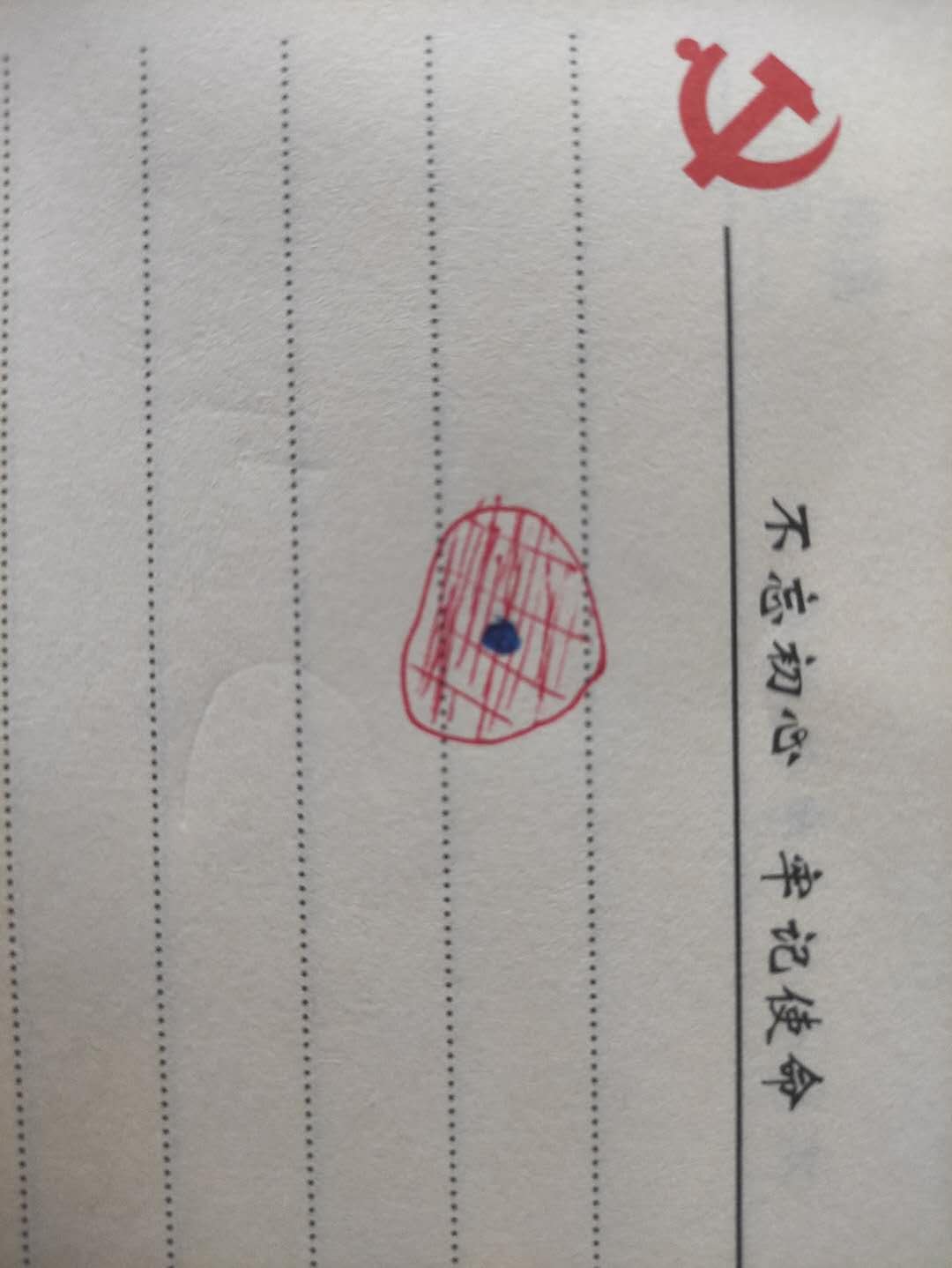这段程序是我从网上看来的，囫囵吞枣的用了，其实没仔细考虑过。

如果从图片的构成来考虑，我一个图是用像素点拼起来的，我能不能仔细去一个识别每个像素点的信息，进而把构成曲线的像素点识别出来呢？

看了下图片读取后的信息，二值化以后的图片信息是一个m*n的矩阵，这个矩阵每个点的值要么是1要么是0。我把是的区域显示出来，果然发现它就是我要的那个曲线。就这样，step2被解决了。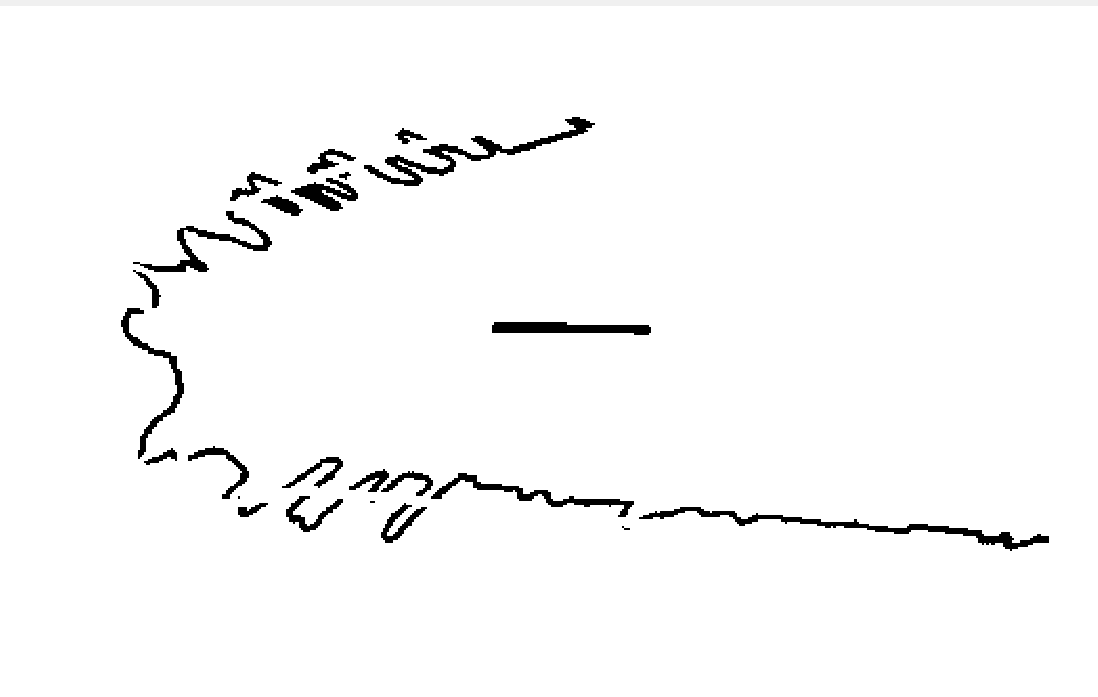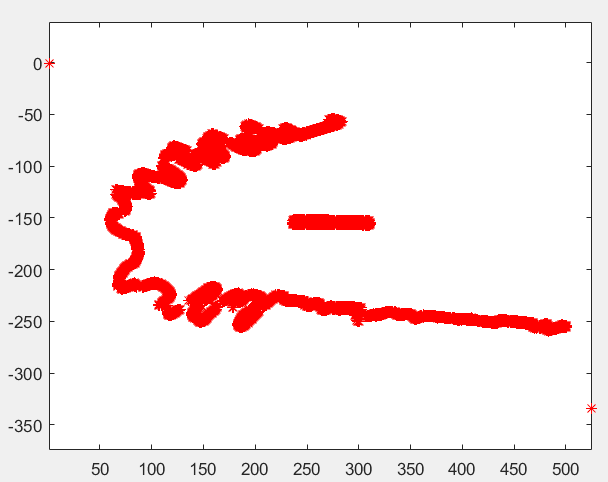2 Step3的解决

现在我的程序可以实现曲线自动提取了，但是我现在提取的坐标是每个像素点在图里的相对位置。比如像素点为100*200，那么我最后一个点的坐标是（100，200）。这显然不能直接用啊，我要知道我图片x、y两个方向的尺度，然后才能换算出真实的坐标值。

我原来解决方案是，在图里面实现加入A、B两个点，通过画图板画上去。

按照我原来的方法，这个还要用画图板，有点麻烦，我觉得应该可以简化。

要简化还是要回到图片上来。像素点是均匀的，如果我知道图片x\y两个方向真实的长度，除以两个方向像素点的个数不就可以确定每个像素点的坐标了吗？

按照这个思路，我图片的实际尺寸我是要实现知道的。但是通常图片都有白边界，因此，需要对图片进行截图处理。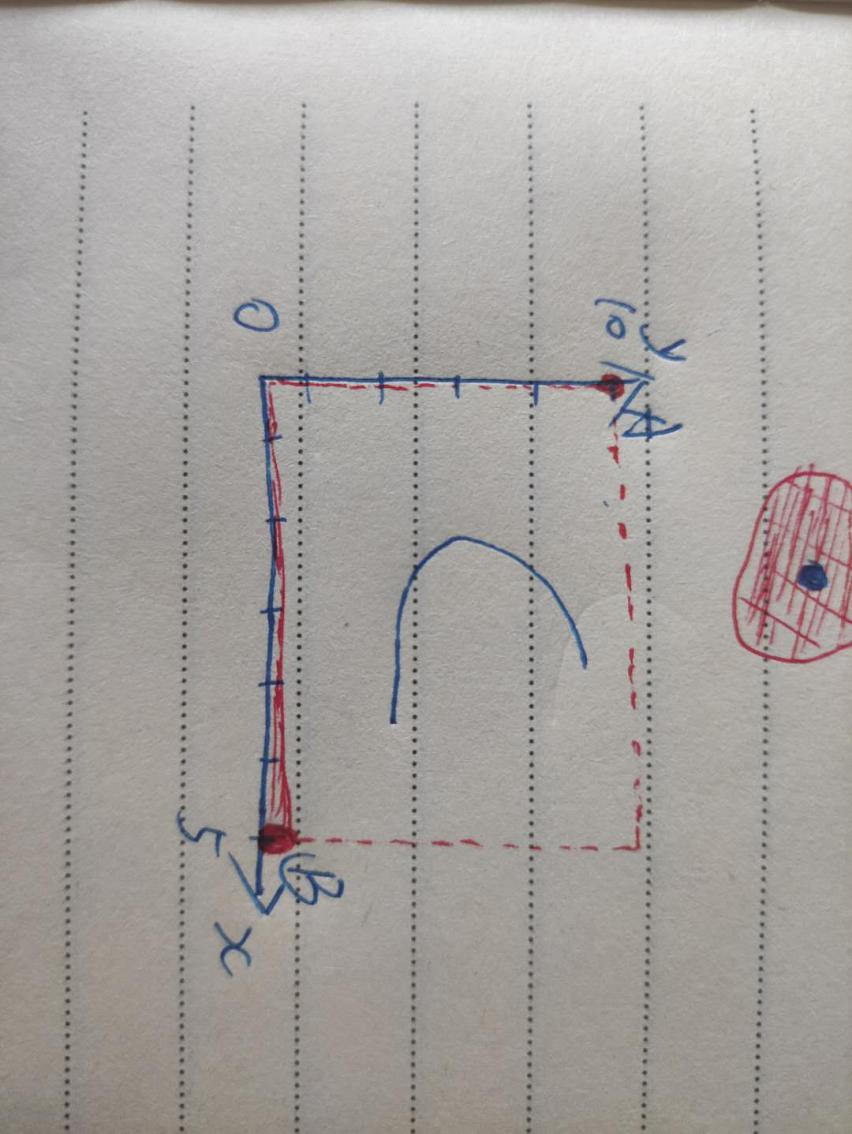根据这个思路，我现在程序效果得到了极大的提升。

3 改进后的效果

改进以后，我现在的程序，分三步：

1）截图。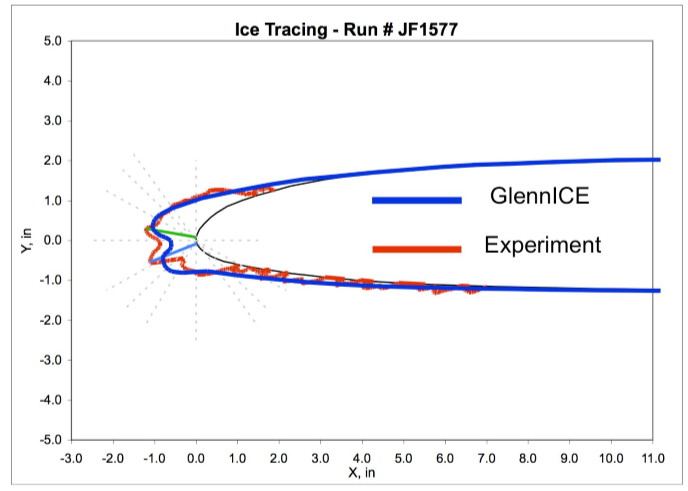截图后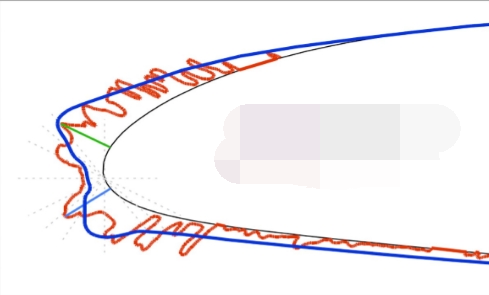3）输入图片两个角点坐标。

4）运行程序。二值化结果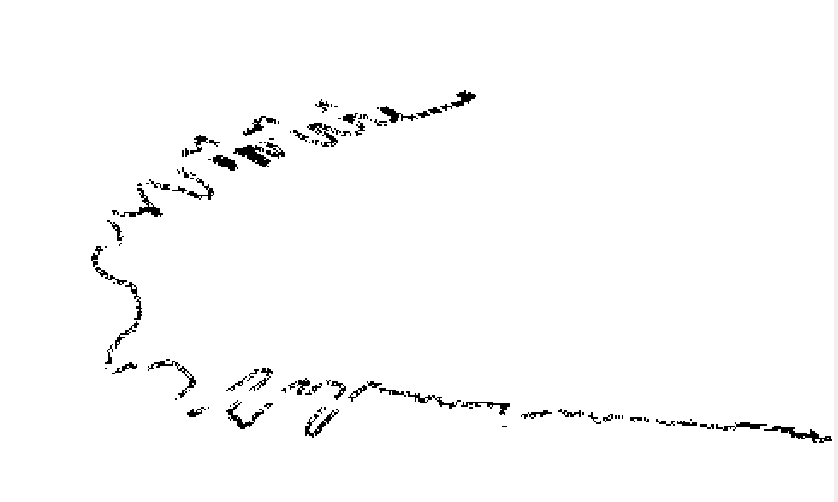坐标结果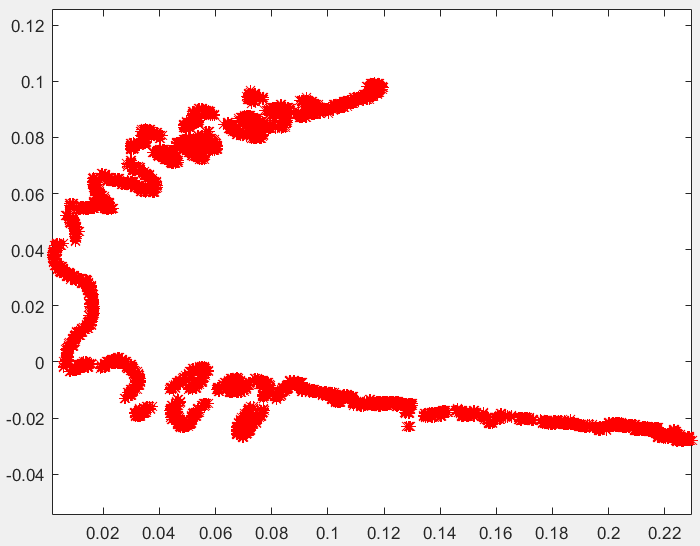最后，半个小时处理完了所有图片，一世英名得以保存。

总体来说，目前这个方法相比于GetData，速度更快，不需要手动点选。另外自动提取像素点，所得结果更密、更准，是一次很好的改进体验。

最后，有需要欢迎通过微信公众号联系我们。

微信公众号：320科技工作室。

展开全文weixin_44873868 2020-09-14 22:00:13
• weixin_39777213 2021-05-07 04:28:26
• Matlab如何用鼠标获取图像的像素值和坐标-test.m matlab

5KB weixin_39840387 2019-08-13 06:03:20
• Matlab如何用鼠标获取图像的像素值和坐标-test.fig matlab

9KB weixin_39840387 2019-08-13 06:03:09
• 13KB qq_40370677 2018-09-28 14:00:56
• weixin_42514709 2021-04-18 03:42:35
• 原创Matlab提取圆点中心坐标-circle.rar matlab

3KB weixin_39840650 2019-08-13 01:06:31
• weixin_29079643 2021-04-18 04:59:55
• weixin_39646412 2021-04-18 02:49:10
•5星
24KB weixin_43723517 2020-11-22 17:58:25
• weixin_31710835 2021-05-04 09:36:17
•5星
2.57MB liuleplayer 2011-10-29 22:25:38
• 【图像识别】图片坐标轴数据自动识别读取matlab代码 matlab 开发语言 算法

qq_59747472 2021-12-12 16:13:42
• qq_36109644 2018-05-23 09:29:01
• weixin_39887531 2020-12-20 17:21:33
• walterchung 2020-09-24 16:44:06
• matlab 提取图像轮廓（图像边缘提取） edge 提取轮廓

Ibelievesunshine 2019-09-01 17:00:06
• MATLAB中操作图像时坐标系中x,y的使用 matlab 图像处理 计算机视觉

qq_15295565 2019-08-17 10:04:16
•5星
1.5MB smjnk 2015-04-17 09:09:44
• csdnxiekai 2021-08-12 16:15:30
• weixin_29531177 2021-04-22 18:10:29
• weixin_33614878 2021-04-18 04:06:09...

matlab提取图像坐标数据matlab 订阅